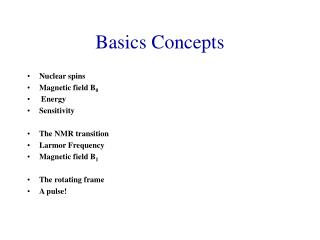DownloadDownload PresentationBasics Concepts

# Basics Concepts

Download Presentation## Basics Concepts

- - - - - - - - - - - - - - - - - - - - - - - - - - - E N D - - - - - - - - - - - - - - - - - - - - - - - - - - -
##### Presentation Transcript

1. Basics Concepts • Nuclear spins • Magnetic field B0 • Energy • Sensitivity • The NMR transition • Larmor Frequency • Magnetic field B1 • The rotating frame • A pulse!

2. E= -m•B0 The energy of the NMR Transition DE=g(h/2p)B0  NUCLEUS • Sensitivity m=-1/2 DE=h0 E m=+1/2 B0 MAGNETIC FIELD B0 Larmor Frequency The two Zeeman level are degenerate at B0=0

3. dm/dt=m(t)^B0(t)

4. M0 B0 M0 = Sm = Mz Mx = My = 0 I=1/2 E = -mB0 E = -gmB0 a: m = +½ ma = +½g Ea = -½gB0 DE = gB0 = w0 = hn0 b: m = -½ mb = -½g Eb = +½gB0

5. Mz=-1/2 hg/2p Mz=1/2 hg/2p w0=2pn0 w0=gB0 n0=gB0/2p E=-mB0 = -ghmzB0/2p DE=ghB0/2p STILL, NO NMR EXPERIMENT

6. A pulse! B1 is a radiofrequency transmitter B1(t)= B1  cos w1t

7. A pulse! Double precession dM(t)/dt= M(t)^B(t) B(t)= B0 + B1(t) dM(t)/dt= M(t)^B0 + M(t)^B1 (t) = M(t)^B0 + M(t)^|B1| cosw1t (t) Precession around B1 (axis defined in the xyplane and rotating at speed w1) Precession around B0 (z axis)

8. Laboratory Frame • Nuclear frequency n1= precession frequency of magnetic field B1 A pulse!

9. The Rotating frame • X’,y’,z’ =laboratory frame • X,y,z,=rotating frame (rotating at the frequency n1) In the rotating frame,there is no frequency precession for m and the radifrequency B1 is seen as a static magnetic field The static magnetic field B0 is not observed in the rotating frame

10. Laboratory Frame Fly A (Laboratory Frame) jam The movement of Fly B as seen by Fly A Fly B Rotating Frame jam Fly B The movement of Fly B as seen by Fly A Fly A (Rotating frame)

11. Precession in the laboratory frame dM/dt=M^B dM/dt=M^(B-w0/g) If B = B0 dM/dt=0 R.F. at freq. w0 L.F. If B = B0+B1 dM/dt=M^(B0 +B1 -w0/g) Rotation! dM/dt=M^B1 = w1g Dw0 If ww0 B1 Dw0 dM/dt=M^(B+B1 -w0/g) dM/dt=M^(B1+(w-w0/g)) dM/dt=M^(B1+(Dw/g))

12. Precession in the laboratory frame Mxy from any nuclear spin not exactly on resonance, will also precess in the x’y’ plane at the difference frequency Dw0. Dw0

13. Basics (II) • Free Induction Decay (FID) • Fourier transformation A pulse

14. Free Induction Decay (FID) Observed NMR signal in the time domain Resonance frequencies are acquired as a function of time t t t Common case of observed FIDs

15. What happens? Relaxation. Magnetization disappear from the xy plane because the system goes back to the equilibrium. The observed signal is always an Exponential DECAY. Chemical shift precession. Different spins may have a different resonance frequency. When the resonance frequency is different from that of the field B1, the signal rotates on the xy plane, with a precession ferquency n1-n0

16. Thank you, Mr. Fourier! F(n) F(t)

17. F(n)=d(n0) F(n)=At(sinpvt)/pvt centered at n0 F(t)=exp(-t/T2) F(n)=T2/1+(2pnT2)2 -i 2pn(T2)2/1+(2pnT2)20 F(t)=exp(-t/T2)exp(i2pnA) F(n)=T2/1+(2p(n-nA)T2)2 -i 2p (n-nA)(T2)2/1+(2p (n-nA) nT2)20 FOURIER TRANSFORMATIONS

18. Why bother with FT? FT allows to decompose a function in a sum of sinusoidal function (deconvolution). In NMR FT allows to switch from the time domain, i.e. the signal emitted by the sample as a consequence of the radiofrequency irradiation and detected by the receiving coil to the frequency domain (NMR spectrum) The FT allows to determine the frequency content of a squared function

19. A “real” F.I.D.

20. Excitation pulses • A single resonance at Larmor frequency n1= excitation frequency n0 (precession of the rotating frame) Transmitter B1 A Pulse B1 on. A pulse! The My magnetization is observed by the receiver coil B1 switched off

21. Received signal • The received signal n1 is compared with the excitation frequency n0 • The resulting signal has observed frequency n=0 • During acquisition time the signal relaxes (T2) My=exp(t/T2) Time (t) Fourier Transformation n=0 Frequency (n)

22. Excitation pulses • A single resonance at Larmor frequency n1different from excitation frequency n0 (precession of the rotating frame) Transmitter B1 Pulse B1 on. A pulse! The My magnetization is observed by the receiver coil B1 switched off

23. Received signal My=cos(nobs)texp(t/T2) • The received signal n1 is compared with the excitation frequency n0 • The resulting signal has observed frequency nobs=(n1-n0) • During acquisition time the signal relaxes (T2) My=cos(nobs)texp(t/T2) Time (t) Fourier Transformation n=0 n=nobs Frequency (n)

24. relax. Preparation Detection z t2 y w0 FT x

25. Absorbance Typical 1H NMR Spectrum

26. Protein 1H NMR spectrum: a “real spectrum” The NMR signal in the time domain Free Induction Decay A short pulse will excite all spins All spins will relax (all together) during time AQ The FT of FID gives the NMR spectrum Fourier Transformation